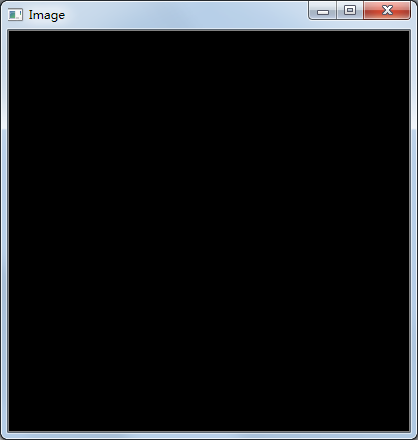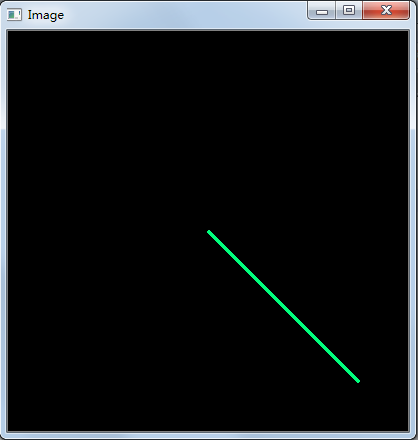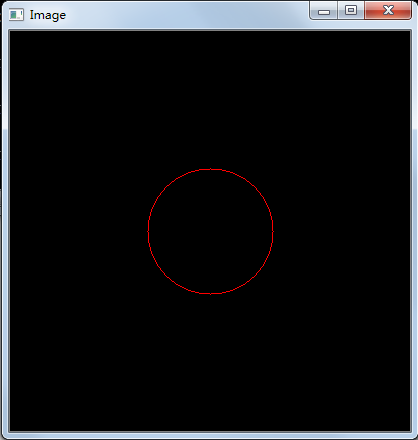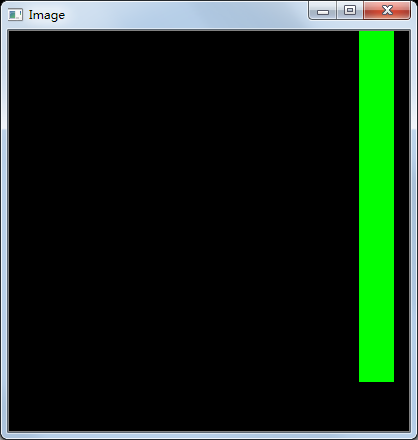# [python opencv 计算机视觉零基础到实战] 十六、用opencv画画1人已赏
1_bit 发布于 2021-01-11 19:30:03 浏览 2627 点赞 79 收藏 6

## 一、学习目标

1. 了解如何使用line方法
2. 了解如何使用rectangle方法
3. 了解如何使用ellipse方法

# 二、了解OpenCV的绘图方法

## 2.1 了解直线绘图方法

``cv2.line(img, pt1, pt2, color[, thickness[, lineType[, shift]]])``
1. 其中img表示需要在哪一张图片上进行绘图
2. pt1表示起始点位置
3. pt2表示终点位置
4. color表示需要绘制的颜色
5. thickness表示需要绘制的线段宽度
6. lineType表示线段的类型，可以为8、 4以及CV_AA shift表示坐标点的小数点位

``````import cv2
import numpy as np

img=np.zeros((400,400,3), np.uint8)``````

``````cv2.imshow("Image", img)
cv2.waitKey (0)
cv2.destroyAllWindows()``````随后我们使用line方法在当前图片上进行绘图。

``cv2.line(img,(0,0),(400,400),(255,0,0),2)``

``cv2.line(img,(0,0),(400,400),(124,252,0),2)``

``cv2.line(img,(200,200),(350,350),(124,252,0),2)``## 2.2 了解矩形绘图方法

``cv2.rectangle(img,(384,0),(350,350),(0,255,0),3)``

## 2.3 了解圆形绘图方法

``cv2.circle(img, center_coordinates, radius, color, thickness)``
• circle方法接收img作为图片参数
• center_coordinates为圆的中心坐标
• color表示绘制圆形的颜色
• thickness表示线段的粗细

``cv2.circle(img,(200,200),63, (0,0,255),1)``那如何进行形状的填充呢？只需要将线段的粗细1改为负一就可以将这个原型给填充颜色。

``cv2.circle(img,(200,200),63, (0,0,255),-1)``

``cv2.rectangle(img,(384,0),(350,350),(0,255,0),-1)````cv2.ellipse(image, centerCoordinates, axesLength, angle, startAngle, endAngle, color [, thickness[, lineType[, shift]]])``
• image为绘制的图像
• centerCoordinates为椭圆的中心坐标
• axesLength有两个变量，包含椭圆长轴和短轴
• angle为椭圆的旋转角度
• startAngle为椭圆弧的起始角度
• endAngle为椭圆弧的终止角度
• color为绘制颜色
• thickness为绘制的线段粗细
• lineType为边界类型
• shift为中心坐标中的小数位

``cv2.ellipse(img, (200, 200), (170, 130), 0, 0, 360, (255, 255, 255), 3)``

## 三、总结

1. 了解使用line方法绘制直线
2. 了解使用rectangle方法绘制矩形
3. 了解使用ellipse方法绘制原型
CSDN博客专家，科技博主，十多年开发经验。

*本文仅代表作者观点，不代表易百纳技术社区立场。系作者授权易百纳技术社区发表，未经许可不得转载。

### 相关专栏海思讨论群新唐科技交流群客服QQ： 1289459644客服手机： 17714209983